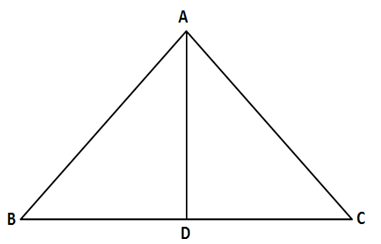• This is an assessment test.
• These tests focus on geometry and mensuration and are meant to indicate your preparation level for the subject.
• Kindly take the tests in this series with a pre-defined schedule.

## Geometry and Mensuration: Test 13

Congratulations - you have completed Geometry and Mensuration: Test 13. You scored %%SCORE%% out of %%TOTAL%%. You correct answer percentage: %%PERCENTAGE%% . Your performance has been rated as %%RATING%%
 Question 1
If the median drawn on the base of a triangle is half its base, the triangle will be:
 A right-angled B acute-angled C obtuse-angled D equilateral
Question 1 Explanation:Since the median is = ½ the base therefore, the triangle has been divided into 2
Isosceles congruent triangles. So each of the triangles is 450-450 -900.
Therefore the sum of the base angles of the original triangle is 450+ 450 = 900.
So the vertex angle must be 90 degrees.
 Question 2
In a right-angled triangle ABC, ∠ABC= 90o, AB=5 cm and BC=12 cm. The radius of the circumcircle of the triangle ABC is
 A 7.5 cm B 6 cm C 6.5 cm D 7 cm
Question 2 Explanation:We know that the radius of the circumcircle = ½ the length of the hypotenuse. The hypotenuse = 13 cm ( by Pythagoras theorem) Thus the required length of the radius = ½ X 13 = 6.5 cm.
 Question 3
The exterior angles obtained on producing the base BC of a triangle ABC in both ways are 120o and 105o, then the vertical ∠A of the triangle is of measure
 A 36o B 40o C 45o D 55o
Question 3 Explanation:The base angles are = (180o-120o) = 60o
and 180-105 = 75o .
Thus the vertical angle = 180o- 60o-75o = 45o.
 Question 4
If AD, BE and DF are medians of ΔABC, then which one of the following statements is correct?
 A $\displaystyle \left( AD+BE+CF \right) B$ \displaystyle AD+BE+CF>AB+BC+CA$C$ \displaystyle AD+BE+CF=AB+BC+CA$D$ \displaystyle AD+BE+CF=\sqrt{2}\,\left( AB+BC+CA \right)$Question 4 Explanation:In an Equilateral triangle , The Height = side x (√3/2) = 0.85 of the side Thus we can conclude that the length of the median < length of the side. Sum of the length of the medians < sum of length of the sides.  Question 5 In a regular polygon, the exterior and interior angles are in the ratio 1: 4. The number of sides of the polygon is  A 10 B 12 C 15 D 16 Question 5 Explanation: The number of sides of the polygon is$ \begin{array}{l}\frac{\frac{360}{n}}{\left( n-2 \right)\times \frac{180}{n}}=\frac{1}{4}\\n=10\end{array}\$
Once you are finished, click the button below. Any items you have not completed will be marked incorrect.
There are 5 questions to complete.
 ← List →